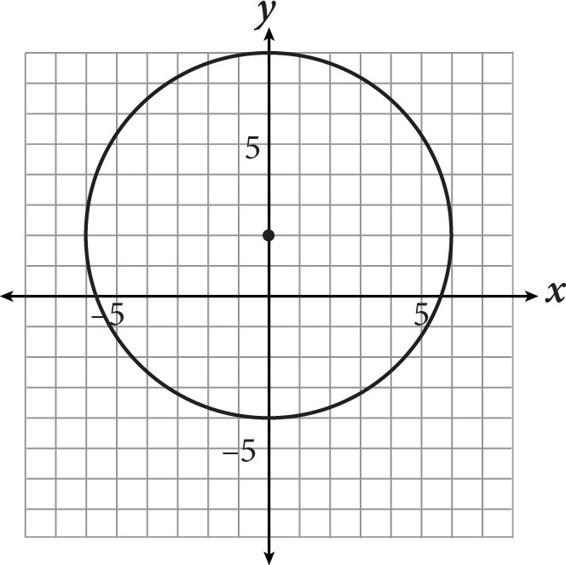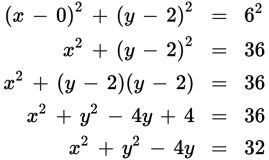# SAT Math Multiple Choice Question 359: Answer and Explanation

### Test Information

Question: 359

14.If the equation of the circle shown above is written in the form x 2 + y 2 + ax + by = c, what is the value of ab + c?

• A. 6
• B. 16
• C. 28
• D. 32

Explanation:

D

Difficulty: Hard

Category: Additional Topics in Math / Geometry

Strategic Advice: Knowing how to write the equation of a circle will earn you points on Test Day. You'll also need to be able to algebraically expand that equation. Don't forget-when you square a binomial, you should write it as repeated multiplication and use FOIL.

Getting to the Answer: First, find the center and the radius of the circle: Each grid-line represents one unit on the graph, so the center is (0, 2), and the radius is 6. Substitute these values into the equation for a circle, (x - h)2 + (y - k)2 = r 2, and then simplify until the equation looks like the one given in the question:There is no x term, so a = 0. The coefficient of y is -4 and c = 32, so ab + c = (0)(-4) + 32 = 32.